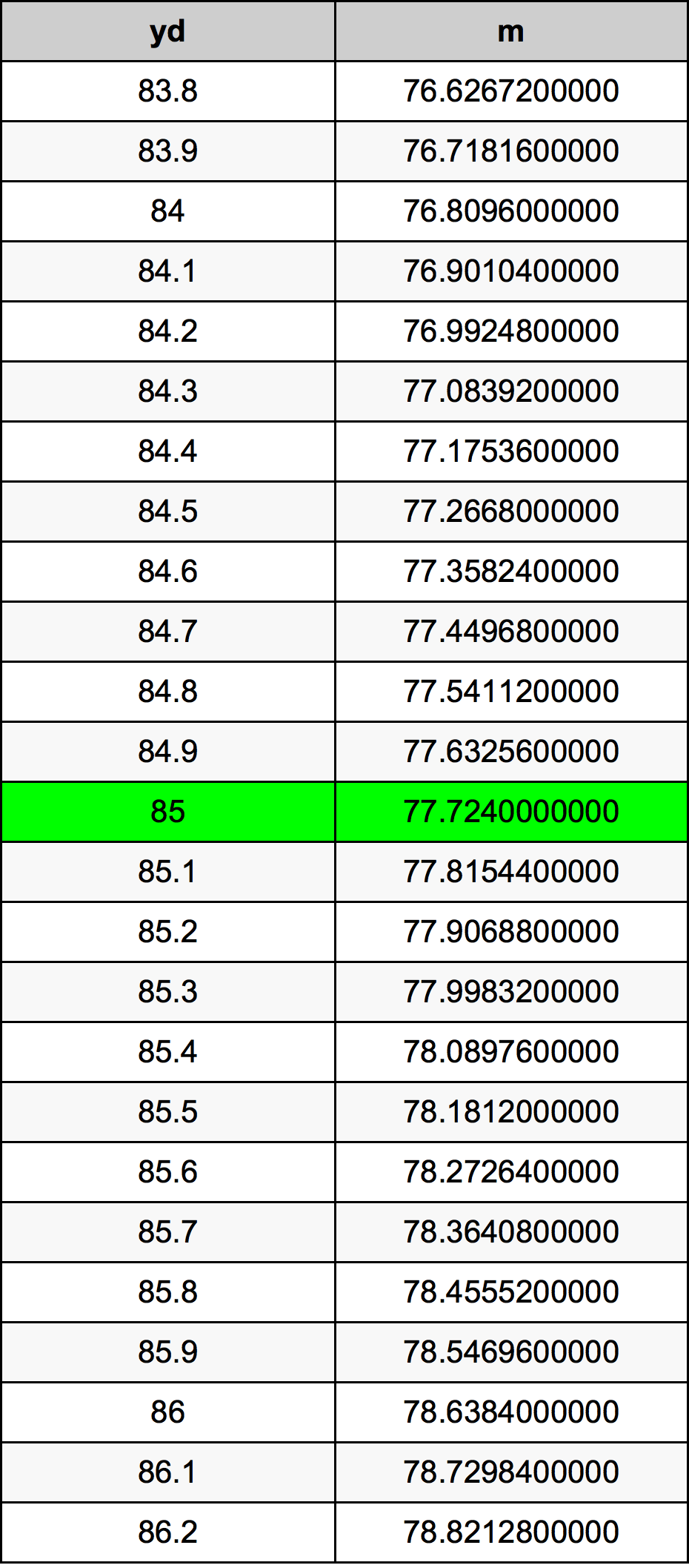Yards To Meters

# 85 yd to m85 Yards to Meters

yd
=
m

## How to convert 85 yards to meters?

 85 yd * 0.9144 m = 77.724 m 1 yd
A common question is How many yard in 85 meter? And the answer is 92.9571303587 yd in 85 m. Likewise the question how many meter in 85 yard has the answer of 77.724 m in 85 yd.

## How much are 85 yards in meters?

85 yards equal 77.724 meters (85yd = 77.724m). Converting 85 yd to m is easy. Simply use our calculator above, or apply the formula to change the length 85 yd to m.

## Convert 85 yd to common lengths

UnitLengths
Nanometer77724000000.0 nm
Micrometer77724000.0 µm
Millimeter77724.0 mm
Centimeter7772.4 cm
Inch3060.0 in
Foot255.0 ft
Yard85.0 yd
Meter77.724 m
Kilometer0.077724 km
Mile0.0482954545 mi
Nautical mile0.0419676026 nmi

## What is 85 yards in m?

To convert 85 yd to m multiply the length in yards by 0.9144. The 85 yd in m formula is [m] = 85 * 0.9144. Thus, for 85 yards in meter we get 77.724 m.

## 85 Yard Conversion Table## Alternative spelling

85 yd to m, 85 yd in m, 85 Yard to Meter, 85 Yard in Meter, 85 Yards to m, 85 Yards in m, 85 yd to Meters, 85 yd in Meters, 85 yd to Meter, 85 yd in Meter, 85 Yards to Meters, 85 Yards in Meters, 85 Yards to Meter, 85 Yards in Meter